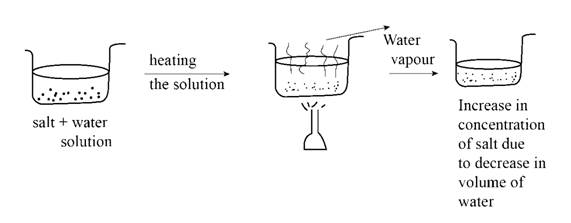Chapter 15, Problem 1ALQIntroductory Chemistry: A Foundati...

9th Edition
Steven S. Zumdahl + 1 other
ISBN: 9781337399425

Solutions

Chapter
SectionIntroductory Chemistry: A Foundati...

9th Edition
Steven S. Zumdahl + 1 other
ISBN: 9781337399425
Textbook Problem

ou have a solution of table sail in water. What happens to the salt concentration (increases, decreases, or stays the same) as the solution boils? Draw pictures to explain your answer.

Interpretation Introduction

Interpretation:

The change in salt concentration as the solution of table salt in water boils is to be stated.

Concept Introduction:

Solution may be defined as the mixture of a minor component which is known as solute and a major component known as solvent. The solute in the solution is uniformly distributed in the solvent. On heating a solution, the liquid generally boils and goes into the air and the salt remains in the remaining solvent as it is nonvolatile.

The concentration of the salt increases as the solution boils.

Explanation

Table salt mixed with water forms a homogeneous solution. When the solution is heated to the boiling point of water, water in the solution boils and goes in the air. Salt in the solution is nonvalatile and does not boils and remain as it is in the solution. Due to this reason, the volume of water decreases and the salt concentration in the solution increases as shown in Figure 1.Figure 1.

Conclusion

The concentration of the salt increases as the solution boils.

The Solution to Your Study Problems

Bartleby provides explanations to thousands of textbook problems written by our experts, many with advanced degrees!

Get Started

Find more solutions based on key concepts